We will talk more about probability distributions in R. The reason why we need to talk about probability and sampling is simple: descriptive statistics (such as the mean and correlation) are derived from a given sample. However, in almost all areas of science, we are interested in estimating those quantities for the general population and not just for the sample at hand. In other words, we need to use inferential tools that allow us to generalize from a given sample to the larger population.

Hopefully you already know some basics of probability from the lectures. Here, I want to introduce how to use the power of simulations to calculate different probabilities.

Throughout this course, we will only deal with five main probability distributions: the binomial distribution, the normal distribution, the chi-squared distribution, the t-distribution and the F-distribution.

# The binomial distribution

Let’s go back to the coin flips. You have two coins (N), and those coins are fair coins. What is the probability of getting one head? let’s enumerate the possible outcomes by the letters H for head and T for tail: HH, HT, TH, TT. So you get one head in two out of four possible outcomes or 2/4 = 1/2 probability.

Now let’s do 3 coins. What is the probability of getting one head? We first enumerate the outcomes: HHH, HHT, HTH, THH, HTT, THT, TTH, TTT. So we have 8 possible outcomes, of which only 3 have one head. So the probability is 3/8.

Binomial distribution works those probabilities for us: we only need to have the number of trials (N: how many events?), and the success probability (P: what is the probability of success in each event?). With those two, we can simply calculate the probability of any event.

Let’s do this using code. In order to get the probability of getting x=1 head, from an experiment of size=2 trials, in which the probability of getting a head on any given trial is p=1/2, we can simply use dbinom:

dbinom(x=1, size= 2, prob = 1/2 )
##  0.5

The probability of getting x=1 head, from an experiment of size=3 trials in which the probability of getting a head in any given trial is p=1/2 will be:

dbinom(x = 1, size = 3, prob=1/2)
##  0.375

The probability of getting 3 heads will be 1/8, since we only have one HHH, or

dbinom(x = 3, size = 3, prob=1/2)
##  0.125

The probability of 2 heads (and one tail: HHT, HTH, THH) will be 3/8 or

dbinom(x = 2, size = 3, prob=1/2)
##  0.375

One final example: let’s do an experiment with size=6 coins, and I want to calculate the probability of getting x=5 heads:

dbinom(x = 5, size = 6, prob=1/2)
##  0.09375

And what is the probability of getting x=5 heads OR x=2 heads? You simply add the two according to the addition rule:

dbinom(x = 5, size = 6, prob=1/2) + dbinom(x = 2, size = 6, prob=1/2)
##  0.328125

So the size argument is the number of trials, while the x argument is the number of outcomes.

## “at least or at most”

Now, let’s shift the question a bit. I have 3 coins, and I want to calculate the probability of getting at least 1 head. Let’s first enumerate the possibilities again:

HHH, HHT, HTH, THH, TTH, THT, HTT, TTT

And this time, I want to calculate how many of those have at least one H. Since I have 7 out of the 8 with at least one head, then the probability is 7/8. However, to compute this with the binomial distribution, you will need to use the summation rule: Probability of getting 0 heads + probability of getting 1 head + probability of getting 2 heads, or:

dbinom(x = 0, size = 3, prob=1/2) + dbinom(x = 1, size = 3, prob=1/2) + dbinom(x = 2, size = 3, prob=1/2)
##  0.875

which is also equivalent to saying: the probability of getting at least one head is:

1 - (the probability of getting 0 heads)

1-dbinom(x = 0, size = 3, prob=1/2)
##  0.875

Before we leave the binomial distribution, I want to discuss pbinom which calculates the probability of getting any number of X or less outcomes. For example, we already calculated the probability of getting at least 1 head in size=3 coin flips. Which means that we have to sum of the probabilities of 1, 2 and 3 heads (as we did before):

pbinom(q = 0, size = 3, prob=1/2, lower.tail = FALSE)
##  0.875

Here, lower.tail = FALSE means that we want to sum the numbers to the right of 0 heads. If we set lower.tail = TRUE and q=1, it will calculate the probability of getting 1 head or less:

pbinom(q = 1, size = 3, prob=1/2, lower.tail = TRUE)
##  0.5

which we can confirm by:

dbinom(x = 0, size = 3, prob=1/2) + dbinom(x = 1, size = 3, prob=1/2)
##  0.5

# Binomial to the Normal

With large N (i.e., more trials), the binomial distribution actually approximate the normal distribution. To see this in action, we first introduce rbinorm which throws random samples from the given distribution. Let’s say I have a fair coin (prob=1/2) and I want R to simulate n=10 coin flips then we can simply write:

rbinom(n=10, size=1, prob=1/2)
##   0 0 0 1 1 1 0 1 0 1

size here refers to the number of trials in each experiment. If I have size=1 it simply means we are only recording the outcome of a single coin flip. If we change the size to 20, it will simulate 20 coin flips and prints the number of heads in each simulation:

rbinom(n=10, size = 20, prob = 1/2 )
##   12  7  9 14 11 12 11  9 10  8

Now, you see that the number of heads in the first simulation is 10, and 12 in the second simulation etc. To illustrate why this approach a normal distribution as you increase your n, you can make a histogram with n=10:

hist(rbinom( n = 10, size = 20, prob = 1/2 ))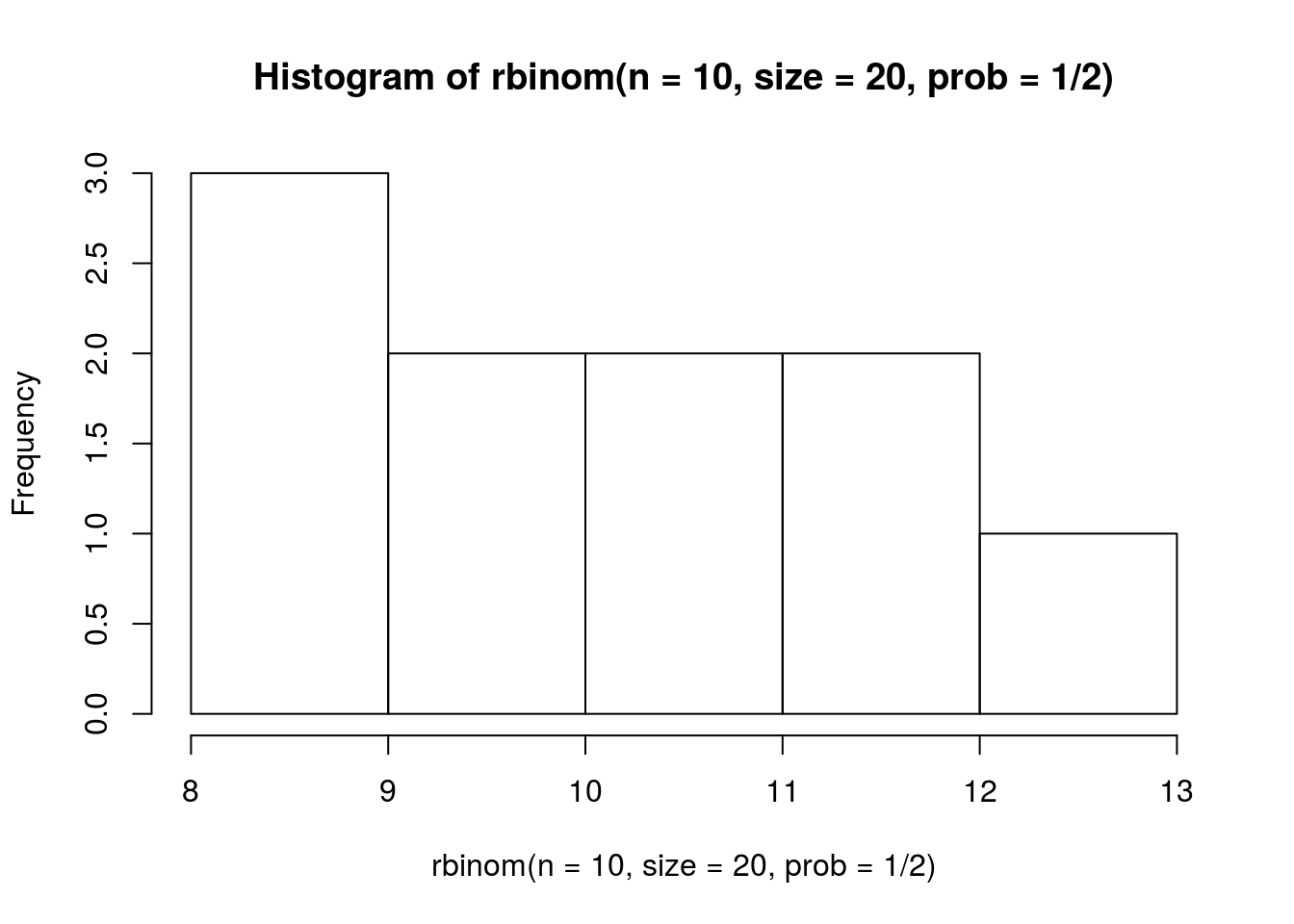and another one with n=1000:

hist(rbinom( n = 1000, size = 20, prob = 1/2 ))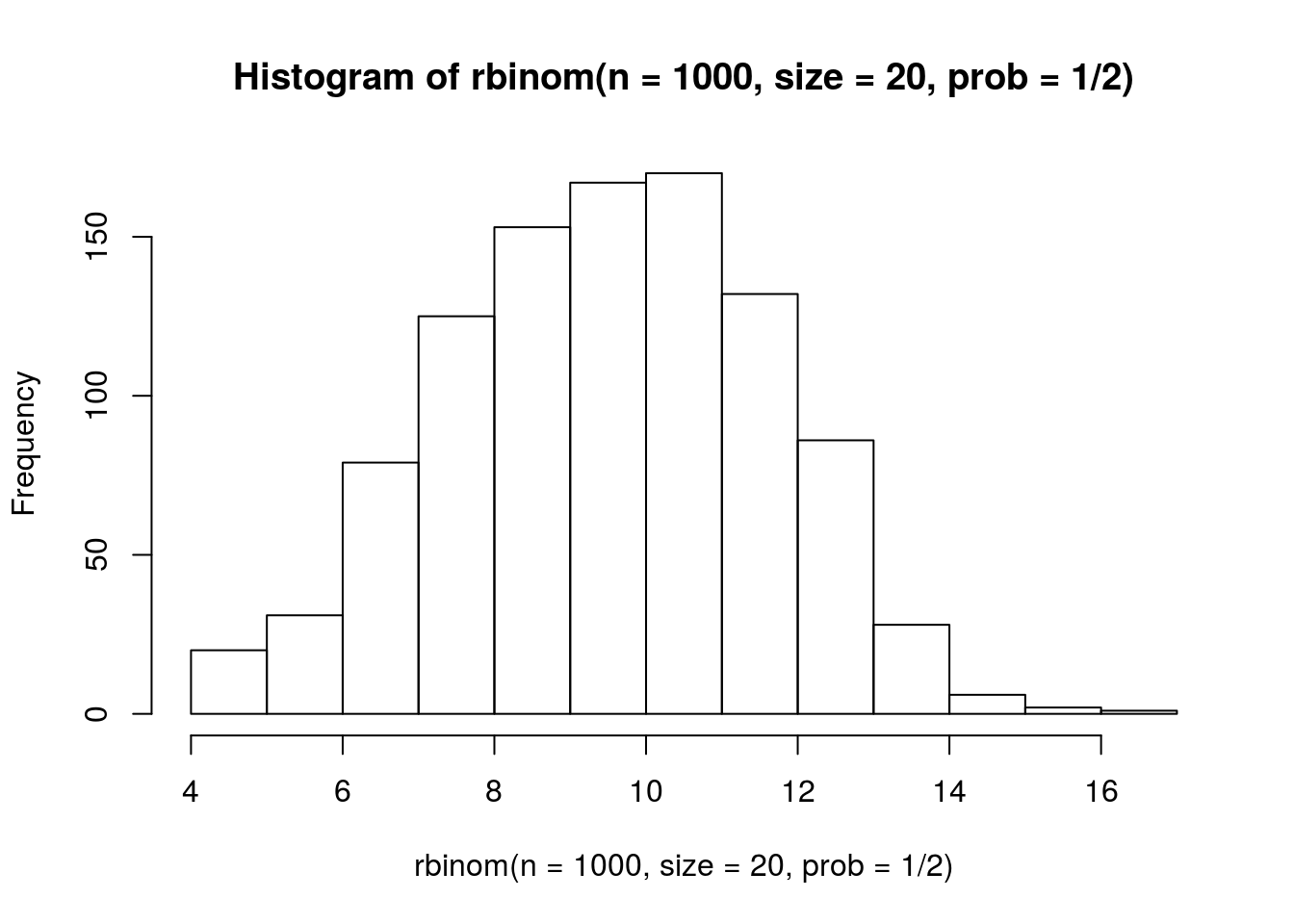The point here is that as you collect more samples from a binomial distribution, you start to approximate the normal distribution.

# The Normal Distribution

Now it is time to talk about the normal distribution, the most important distribution in statistics and probably in life. Normal distribution (or the “bell-curve”) can be described by only two numbers: the mean and the standard deviation from the mean. Similar to the binomial distribution, we have: dnorm(), pnorm(), qnorm() (which I have been ignoring) and rnorm(). The big difference between normal distribution and binomial distribution is that the normal distribution is based on continuous numbers, and not just discrete events. In the binomial distribution, we can’t calculate the probability of getting 1.3 heads in 3 coin flips (you technically can but R will round that for you). In normal distribution, however, we can calculate the probability of any number in the distribution once we know the mean and the standard deviation.

Let’s assume we have a normal distribution of mean=0 and sd=1. First, we can make a nice picture by getting the probability density function at each value between -4 and 4:

x <- seq(-4, 4, length=100)
hx <- dnorm(x)
mydf <- data.frame(x, hx)

library(ggplot2)
ggplot(data=mydf, aes(x=x, y=hx)) + geom_line()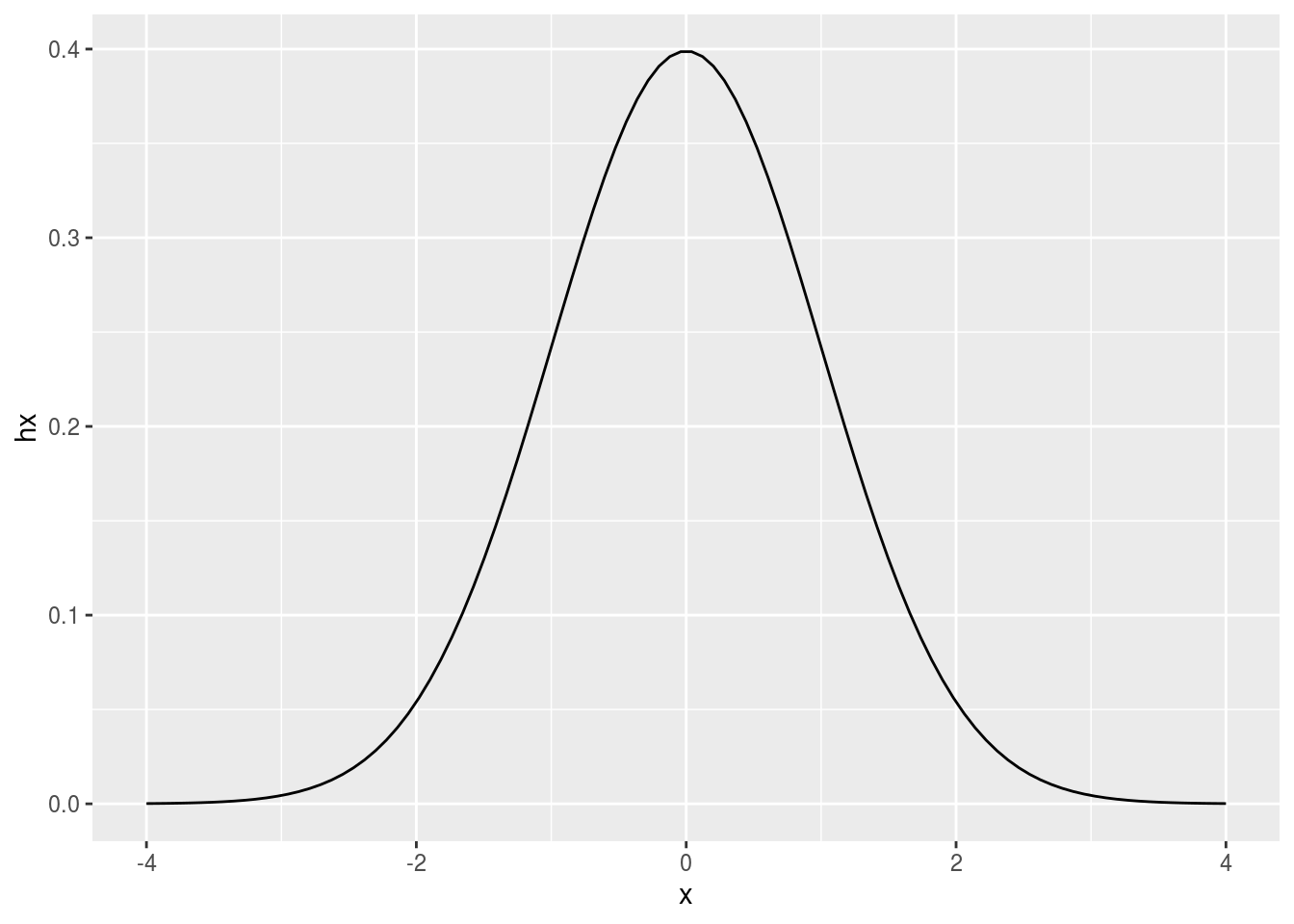Now that we have a normal distribution in front of us, we can calculate the probability of any value (by calculating the size of the area under the curve). Let’s calculate P(X< 0), or what is the proportion of the area that lies below 0?

pnorm(0, mean = 0, sd = 1, lower.tail=TRUE)
##  0.5

Oh, that means half of the area is below 0. Now we can calculate the proportion of area above 1 (and notice lower.tail=FALSE):

pnorm(1, mean = 0, sd = 1, lower.tail=FALSE)
##  0.1586553

wihch means ~15.86% of the area are above 1.

Finally, we can calculate the area between any two points by substracting the two. Let’s calculate the area between 1 and -1:

pnorm(1, mean = 0, sd = 1, lower.tail=TRUE)- pnorm(-1, mean = 0, sd = 1, lower.tail=TRUE)
##  0.6826895

Meaning that ~68% of observations should lie between those two numbers (if numbers were coming from this distribution). Well, we can actually test this using simulations with rnorm. rnorm give us a list of random samples from a given distribution. For example, we can ask for n=10 numbers coming from a normal distribution with a mean of 0 and a standard deviation of 1:

rnorm(n=10, mean=0, sd=1)
##    0.4057008 -1.8783643 -0.8750538  0.1022850 -1.0495061  0.1335285
##    0.2636835 -0.1329724 -2.7522190  1.1509629

Let’s test if ~68% of numbers should lie between -1 and 1 (or if any given number is bigger than -1 and less than 1:

numbers <- rnorm(n=10, mean=0, sd=1)
mean(numbers > -1 & numbers < 1)
##  0.6

I doubt if you will get 0.68! Why is that? because we have too few numbers to play with. If we increase the sample, we will start to get to the true proportion

numbers <- rnorm(n=10000, mean=0, sd=1)
mean(numbers > -1 & numbers < 1)
##  0.6839

We’ll later come back to this. Let’s continue with some practical problems:

IQ scores are normally distributed with a mean of 100 and a standard deviation of 15:

What percentage of people have an IQ less than 125?

pnorm(125, mean = 100, sd = 15, lower.tail=TRUE)
##  0.9522096

What percentage of people have an IQ greater than 110?

pnorm(110, mean = 100, sd = 15, lower.tail=FALSE)
##  0.2524925

What percentage of people have an IQ between 110 and 125?

pnorm(125, mean = 100, sd = 15, lower.tail=TRUE) - pnorm(110, mean = 100, sd = 15, lower.tail=TRUE)
##  0.2047022

We can also use qnorm to find the percentiles. For example, let’s find the value that separates the buttom 15.8% of observations:

qnorm(.158, mean=0, sd=1, lower.tail = TRUE)
##  -1.002712

Or the top 15.8% observations?

qnorm(.158, mean=0, sd=1, lower.tail = FALSE)
##  1.002712

Continuing with our example of IQ, we can ask what IQ score that separates the bottom 25% from the others:

qnorm(.25, mean = 100, sd = 15, lower.tail = TRUE)
##  89.88265

# Sampling , one more time

We just tested if it is true that ~68% of the numbers drawn from a normal distribution with a mean of 0 and a standard deviation of 1 should lie between -1 and 1. We observed that we get slightly different answers. The reason is because in those simulations, we use random sampling. Random sampling means that each number have the same probability to be picked. If I randomly sample an item from a set of 6 items, then each item has the same probability of being sampled, 1/6. The same happens with sampling continuous numbers from a normal distribution using rnorm. In all scientific experiments, we deal with samples from the real world. We almost never deal with the populations. However, as I explained in the beginning, we are interested in making inferences about the populations. Let’s say that IQ test is created so that you have a mean of 100 and a standard deviation of 15. That’s something like high up in the sky. When you, a humble human being, want to measure the average IQ scores (and you don’t know the true mean and standard deviation), you collect a sample. You hope that the average of the IQ score of this sample will approximate the true above-the-sky population average. Let’s first make a sample of 5 IQ scores:

iq_small_sample <- round(rnorm(n=5, mean=100, sd=15))
hist(iq_small_sample)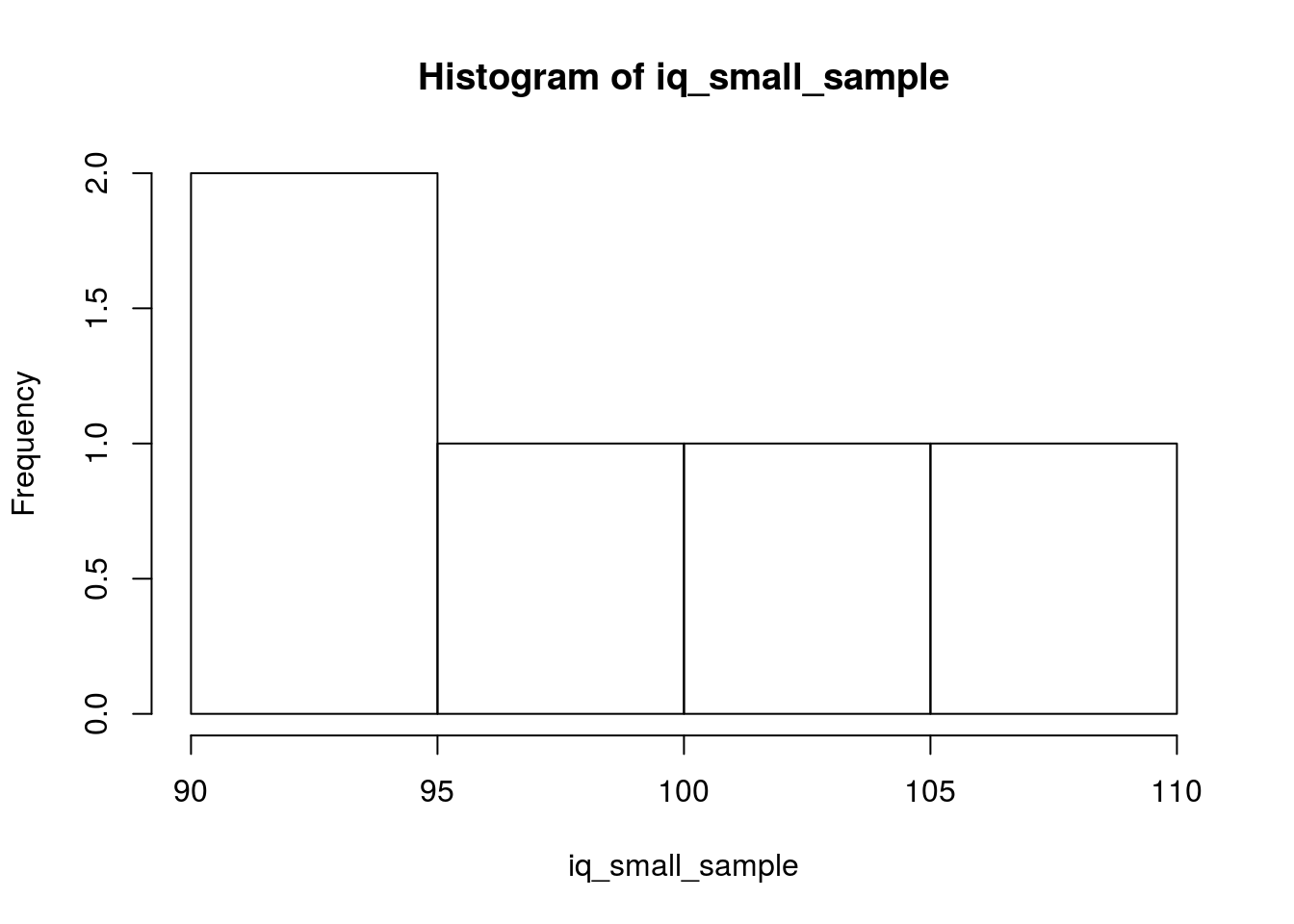if you calculate the average and the standard deviation of the sample you have you will get:

mean(iq_small_sample)
##  99
sqrt(sum((iq_small_sample-mean(iq_small_sample))^2)/(length(iq_small_sample))) # population but divide by N
##  7.042727

Which are slightly different from the true values. Well, the mean is actually much closer to the true mean but the standard deviation is quite far. Let’s get another sample but this time we’ll make it bigger:

iq_moderate_sample <- rnorm(n=30, mean=100, sd=15)
hist(iq_moderate_sample)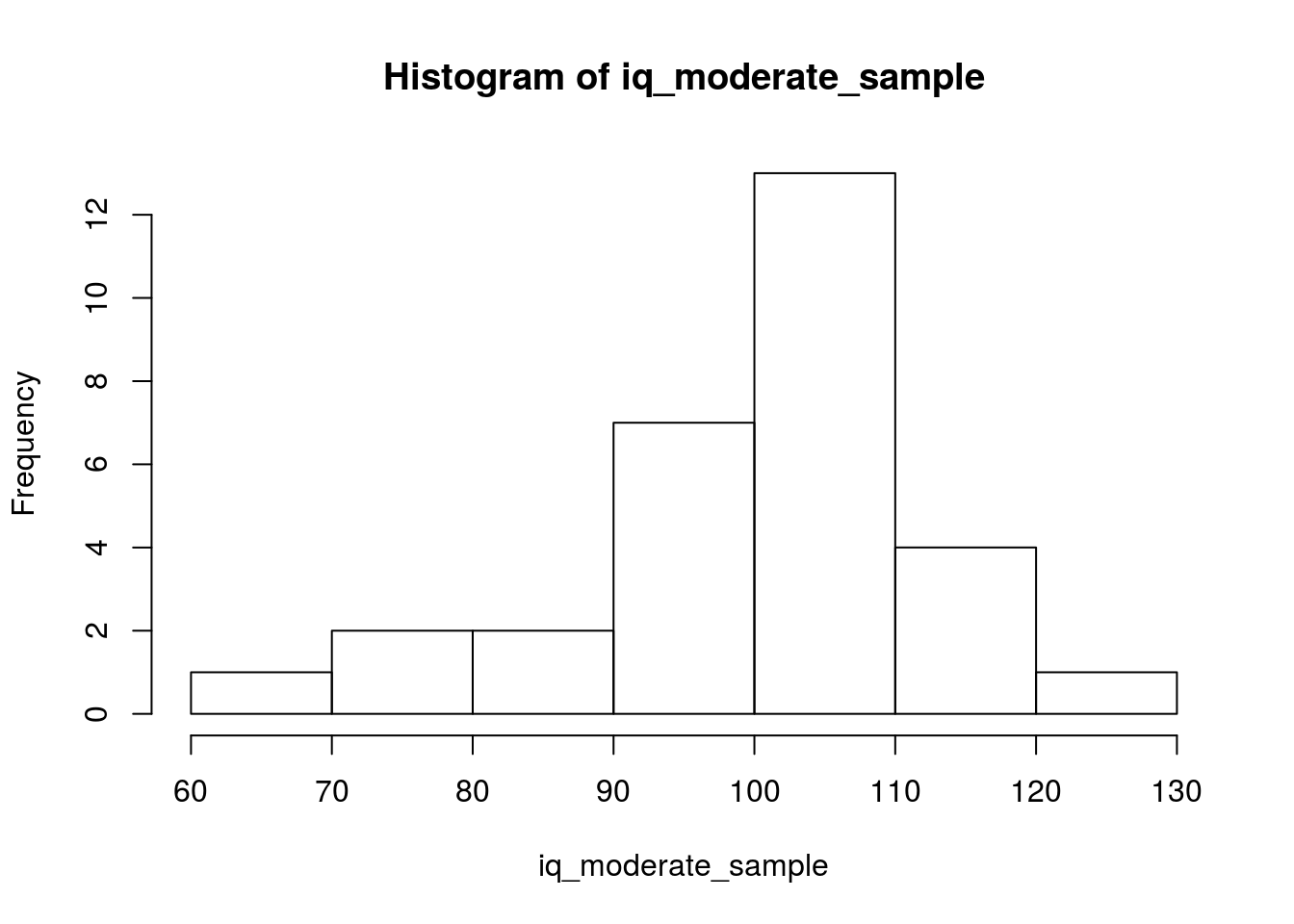mean(iq_moderate_sample)
##  100.6285
sqrt(sum((iq_moderate_sample-mean(iq_moderate_sample))^2)/(length(iq_moderate_sample)))
##  11.91425

And now we got much closer to the true values. Finally let’s make it a very large sample:

iq_laaarge_sample <- rnorm(n=10000, mean=100, sd=15)
hist(iq_laaarge_sample)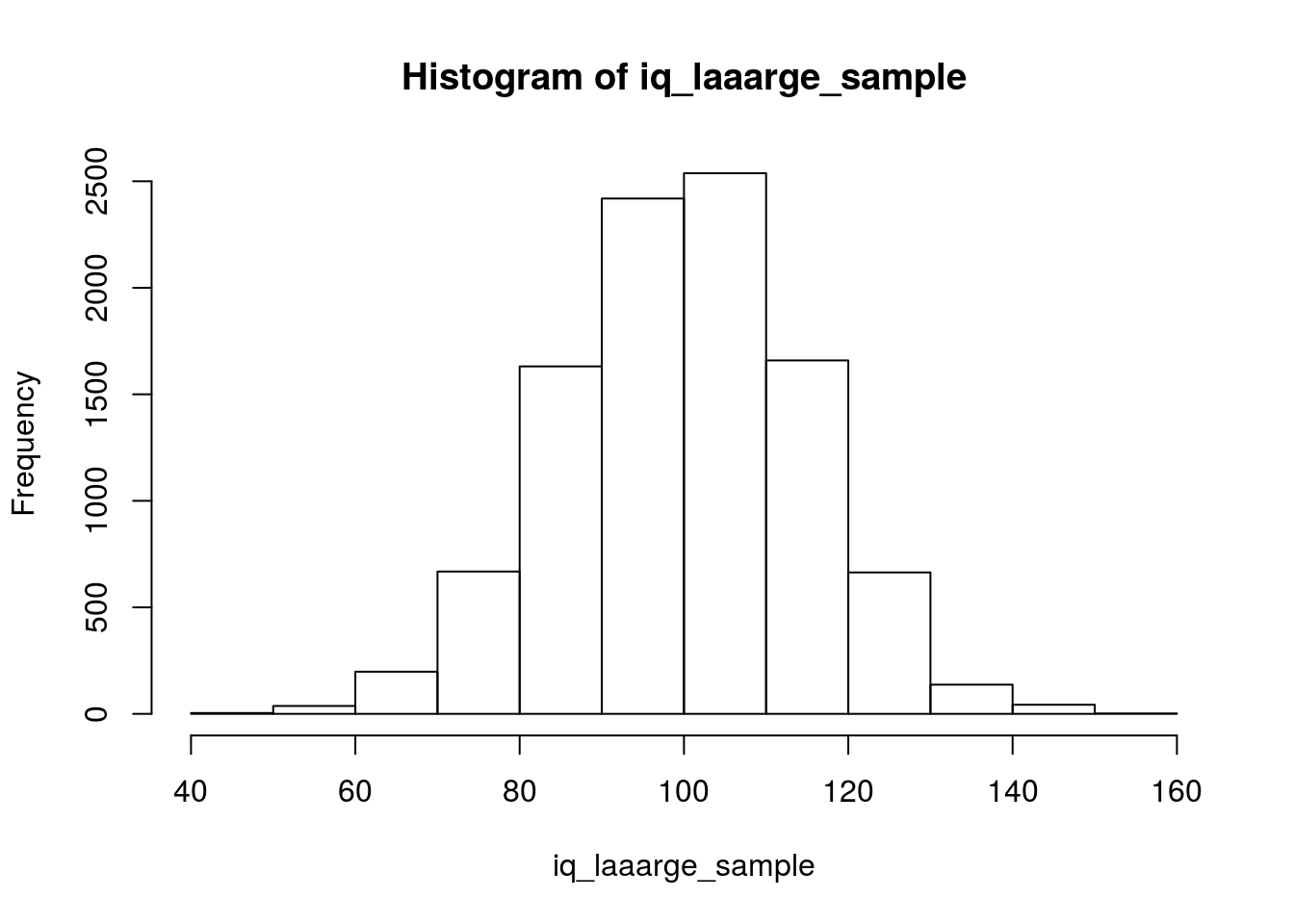mean(iq_laaarge_sample)
##  99.9059
sqrt(sum((iq_laaarge_sample-mean(iq_laaarge_sample))^2)/(length(iq_laaarge_sample)))
##  14.87981

And finally with larger sample, you get very close to the true values of the population mean and standard deviation.

What is happening? Why does the mean is much closer to the true population even with small sample sizes, but the standard deviation needs a larger sample size? The mean is an unbiased estimate of the population mean and that’s why the sample mean is used everywhere – but the sample standard deviation is a biased estimate of the population standard deviation (basically, it is always smaller) and that’s also the reason you divide the standard deviation by N-1 and not by N to correct for this and make the value a bit larger. sd actually use the N-1 formula, so let’s use it and compare its result with our old value:

sqrt(sum((iq_small_sample-mean(iq_small_sample))^2)/(length(iq_small_sample)))
##  7.042727
sd(iq_small_sample)
##  7.874008

How about a little more systematic experiment in R? Let’s do the same calculations but for a range of values of sample sizes and then see how the mean and the standard deviations change as we increase the sample size.

n_experiments <- 1000 # we will conduct X experiments each time
n_samples <- c(1:15) # we will start with a sample size of 1 until X
means_of_sample_n <- c()
sds_uncorrected_of_sample_n <- c()

for (this_sample_n in n_samples) {
this_sample_means <- c()
this_sample_sds_uncorrected <- c()
for (i in 1:n_experiments) {
iq_sample <- rnorm(n=this_sample_n, mean=100, sd=15)
this_sample_means[i] <- mean(iq_sample)
this_sample_sds_uncorrected[i] <- sqrt(sum((iq_sample-mean(iq_sample))^2)/(length(iq_sample)))
}
means_of_sample_n[this_sample_n] <- mean(this_sample_means)
sds_uncorrected_of_sample_n[this_sample_n] <- mean(this_sample_sds_uncorrected)
}

Now we’ll use ggplot to make some pretty visualizations:

#install.packages('ggpubr')
library(ggpubr)

results <- data.frame(n=n_samples,
means=means_of_sample_n,
sds=sds_uncorrected_of_sample_n)

g1 <- ggplot(results, aes(x=n, y=means))+
geom_line(color='red') +
geom_point() +
geom_hline(yintercept = 100) +
ylim(c(95, 105)) +
theme_classic()
g2 <- ggplot(results, aes(x=n, y=sds))+
geom_line(color='red') +
geom_point() +
geom_hline(yintercept = 15) +
ylim(c(-1, 17)) +
theme_classic()

ggarrange(g1, g2, ncol = 2, nrow = 1)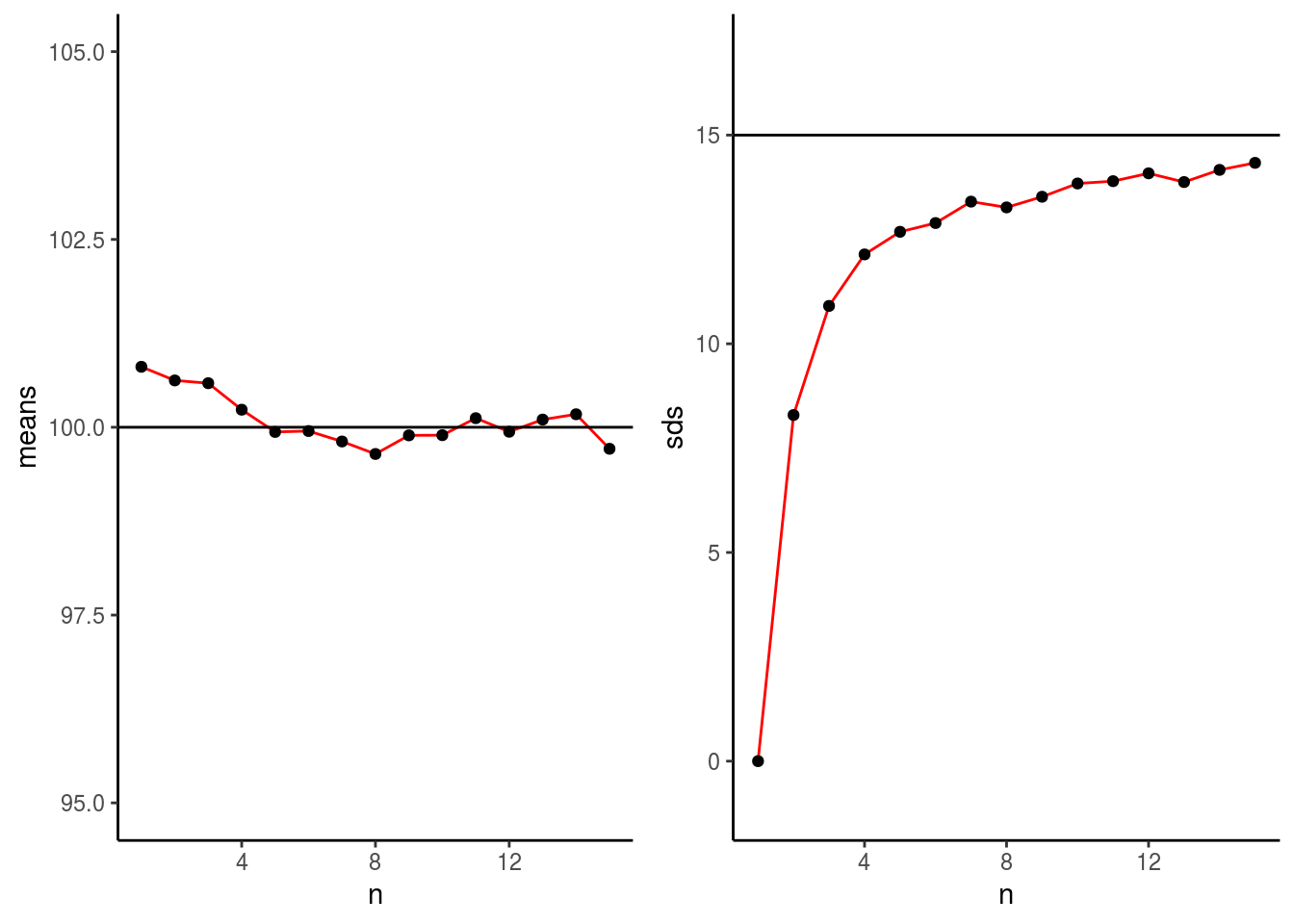Here I will stop for today.

# Exercises

A student is taking an exam with 20 MCQ questions, where each question has 4 choices and the student is randomly answering those questions (so P=0.25). Use R code to answer the following questions:

• Calculate the probability of getting 1 questions correct?

• Calculate the probability of getting AT LEAST 5 questions correct?

• Calculate the probability of getting 15 questions correct?

• Calculate the probability of passing the exam (passing grade is 60%).

• Simulate a class of 100 students taking the same exam (where again each student is making random guesses). What is the average of the class (or the average of correct answers per student)?

• If we increase the class size to 1000 students, what happens to the average? (keep in mind that the true average of the correct answers for any given number of students is simply P * N or 0.25 * 20 = 5 correct answers).

Use simulations to answer the following questions:

• Is the maximum (max) a biased estimate or an unbiased estimate of population maximum?

• Is the median (median) a biased estimate or an unbiased estimate of the population median?

• Is the variance a biased or unbiased estimate of population variance? (DO NOT USE var, rather, use the original formula that divides by N)

You might want to use an R Markdown file to answer those questions.